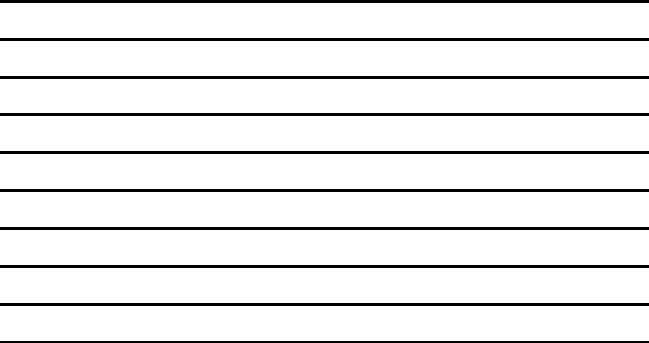## Cdltrucker Answers Test Printable Details

The Cdl Hazmat Test is a certification that you need in order to transport hazardous materials. This test is an extensive examination of knowledge, skills and abilities that drivers must have before transporting hazardous materials by road or rail. It is important for drivers to know the do's and don'ts of transportation because it can be life-threatening if mistakes are made. The Cdl Hazmat Test contains four sections: General Knowledge; Transportation Regulations; Dangerous Goods Handling Procedures; and Roadside Emergencies. The general knowledge section includes questions about driving laws, emergency procedures, vehicle inspections, cargo loading/unloading methods and more.

You'll discover info about the type of form you want to complete in the table. It will tell you how long it may need to complete cdl hazmat test, what fields you need to fill in, and so forth.

Form NameCdl Hazmat Test
Form Length1 pages
Fillable?No
Fillable fields0
Avg. time to fill out15 sec
Other nameshazmat endorsement study guide pdf, cdl hazmat study guide pdf, cdltrucker answers test printable, hazmat printable practice test and answers

## Form Preview ExamplePRINTABLE ANSWERS - CDL HAZMAT PRACTICE TEST - CDLTRUCKER.NET

CDL HAZMAT PRACTICE TEST ANSWERS - CDLTRUCKER.NET

CDLTrucker.net

Page 1:  = a  = d  = a  = b  = a  = a  = d

Page 2:  = a  = b  = c  = d  = b  = c  = c

Page 3:  = a  = d  = b  = a  = c  = d  = b

Page 4:  = c  = c  = c  = d  = b  = c  = d

Page 5:  = d  = b  = c  = b  = d  = b  = a

Page 6:  = b  = b  = c  = d  = b  = a  = d

Page 7:  = c  = a  = a  = a  = b  = b  = c

Page 8:  = a  = c  = a  = c  = d  = c  = d

Page 9:  = d  = c  = a  = a  = c

 CDL HAZMAT PRACTICE TEST ANSWERS - CDLTRUCKER.NET 1